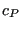## *VALUES AT INFINITY

Keyword type: model definition

This keyword is used to define values at infinity for 3D fluid calculations. They are used to calculate the pressure coefficientif requested as output by the user (*NODE FILE) and freestream boundary conditions for the turbulence parameters .

First line:

• *VALUES AT INFINITY

Second line:

• Static temperature at infinity
• Norm of the velocity vector at infinity
• Static pressure at infinity
• Density at infinity
• Length of the computational domain

Example:

*VALUES AT INFINITY
40.,1.,11.428571,1.,40.


specifies a static temperature of 40., a velocity of 1., a static pressure of 11.428571 and a density of 1. at infinity. The size of the computational domain is 40.

Example files: fluid1,fluid2.